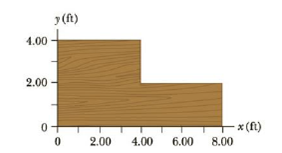Chapter 8, Problem 11P

Chapter
Section
Textbook Problem

Find the x- and y-coordinates of the center of gravity of a 4.00-ft by 8.00-ft uniform sheet of plywood with the upper right quadrant removed as shown in Figure P8.ll. Hint: The mass of any segment of the plywood sheet is proportional to the area of that segment.Figure P8.11

To determine
The x -and y -coordinates of the center of gravity of a 4.00ft by 8.00ft uniform sheet of plywood with the upper right quadrant removed.

Explanation

Section1:

To determine: The x -coordinate of the center of gravity of a 4.00ft by 8.00ft uniform sheet of plywood with the upper right quadrant removed.

Answer: The x -coordinate of the center of gravity of a 4.00ft by 8.00ft uniform sheet of plywood with the upper right quadrant removed is 3.33ft .

Explanation:

Given info: The area of the part A1 of the plywood is 16.0ft2 , the area of the part A2 is 8.00ft2 , and x coordinates of the center of gravity of parts of the plywood A1 , A2 are 2.00ft and 6.00ft .

Assume the remaining parts of plywood as two parts A1 with an area of 4.00ft by 4.00ft and its center of gravity is located at (2.00ft,2.00ft) . Similarly, another part of the plywood is A2 with area of 2.00ft by 4.00ft and the location of the center of gravity of this part is (6.00ft,1.00ft) . The mass of the plywood is proportion to its area; its mass is defined as m=σA where σ is proportionality constant. In this way, the masses of each part can be determined.

The formula for the x co-ordinate of the center of gravity is,

xcm=ρA1x1+ρA2x2ρA1+ρA2

• ρ is proportionality constant.
• A1 and A2 are areas of the plywood respectively.
• x1 and x2 are the x -coordinates of the center of gravity of the parts of plywood.

Substitute 16.0ft2 for A1 , 8.00ft2 for A2 , 2.00ft for x1 , and 6.00ft for x2 to find xcm .

xcm=ρ(16.0ft2)(2.00ft)+ρ(8.00ft2)(6.00ft)ρ(16.0ft2)+ρ(8.00ft2)=3.33ft

Thus, the x -coordinate of the center of gravity of a 4

Still sussing out bartleby?

Check out a sample textbook solution.

See a sample solution

The Solution to Your Study Problems

Bartleby provides explanations to thousands of textbook problems written by our experts, many with advanced degrees!

Get Started

Find more solutions based on key concepts